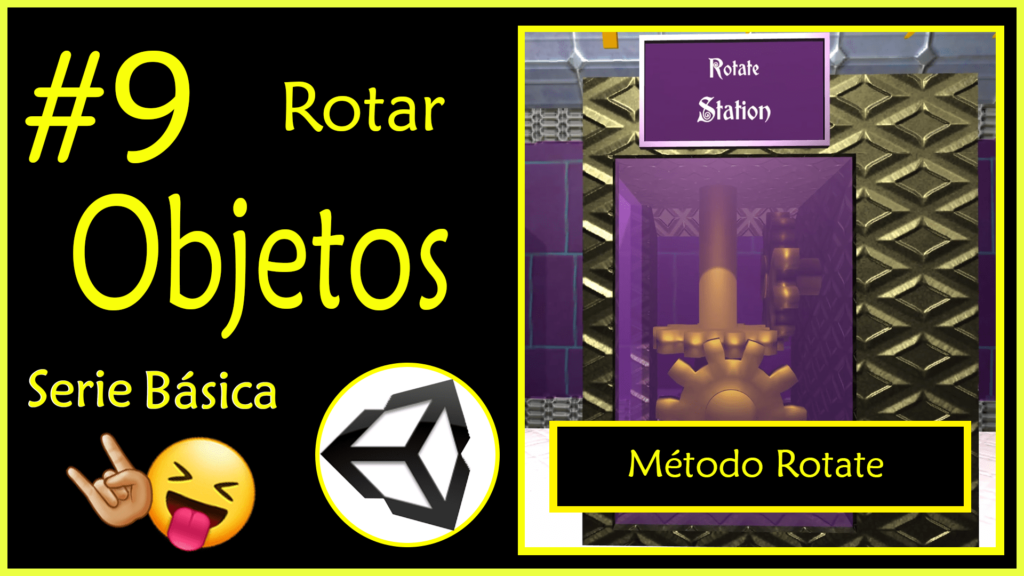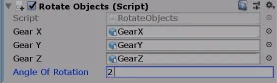# #9 Rotate objects in Unity. Rotate method of the Transform class.

## Introduction

In this article we’re going to see how to rotate objects in Unity. There are several ways to achieve this, in this case we will use the Rotate method of the Transform class, this will directly modify the rotation parameters that are observed at the top of the inspector with the GameObject selected.

## Go to the project’s main page

Before we start I invite you to watch the video I made to summarize this article. English Subtitles available.

## How are rotations expressed mathematically?

In order to describe mathematically we have to know directly or indirectly the following parameters:

-Rotation axis

-Amount of angles to rotate

-Sense of rotation

Let’s imagine that the object we want to rotate is a sphere. We take it in our hands and stick a rod through the exact center of the sphere. Then we take the rod and orient it from north to south parallel to the ground. Finally we make it turn counterclockwise.

### Were you able to visualize it in your mind?

My description of the rotation was accurate although perhaps it was not drafted in the best way to be understood. However, let us assume that we could all have imagined exactly the same thing.

In the first place we can say that the axis of rotation is the rod. If we become strict the axis of rotation is an imaginary line that passes through the two ends of the rod.

The number of angles to rotate is half a turn, this mathematically is 180° or π radians.

The direction of rotation is counterclockwise or counterclockwise.

These three parameters can be fully described using a vector, which is what we are going to do in this article.

## Workstation

We are going to use the rotation station which consists of three gears that are oriented in the direction of the world’s three x,y,z axes.

The three gears are positioned perpendicular to each other.

In the hierarchy we can find them inside the GameObject “#9 RotateObjects”. They have the names GearX, GearY and GearZ indicating the axis of rotation of each gear.

The script to complete is called “RotateObjects” and is assigned to GameObject with the same name in the hierarchy.

In figure 4 we see that the three GameObjects of the hierarchy are already assigned in the fields of the inspector.

When opening the Script we find the FixedUpdate method defined and inside some comments that say that the gears must be rotated there.

If it is not clear what a method is in my channel there is a video in which I talk about methods in programming (english subtitles available) and there is also an article in the programming section of this page.

### Why should the rotation code go in the FixedUpdate method?

In the Article 1 about reading keyboard and mouse inputs we discussed why reading instructions were in the Update method.

The FixedUpdate method as its name indicates is an update of the object state but executed at a certain fixed time.

This means that no matter how much the frame rate of the game changes, we will have the same number of executions of this method per second.

Colocaremos en el método FixedUpdate una instrucción que hará que los engranajes giren una determinada cantidad de ángulos en algún sentido respecto de un eje. Esto significa que en cada llamada de FixedUpdate se aplicará la rotación, lo que generará un movimiento continuo de rotación.

## Solution

The Rotate method of the Transform class has different versions, we are going to use one in which the first parameter is the vector that completely expresses the rotation and in the second parameter we indicate the type of coordinates to use, global coordinates that are unique in the whole world of the game or local coordinates that are specific to each particular object, which would be as if each object had its own north.

By mathematical properties of the vectors I rewrote the vector as the product of its module or norm multiplied by a unit vector (norm 1) describing the direction.

This allows us to separate on the one hand the direction of rotation and on the other hand the number of angles to rotate.Fig. 8: Modifying the variable in the inspector we can change the speed of the set of gears.

Another advantage of expressing the vector that way is that we can change the direction of rotation simply by using negative values in the inspector.